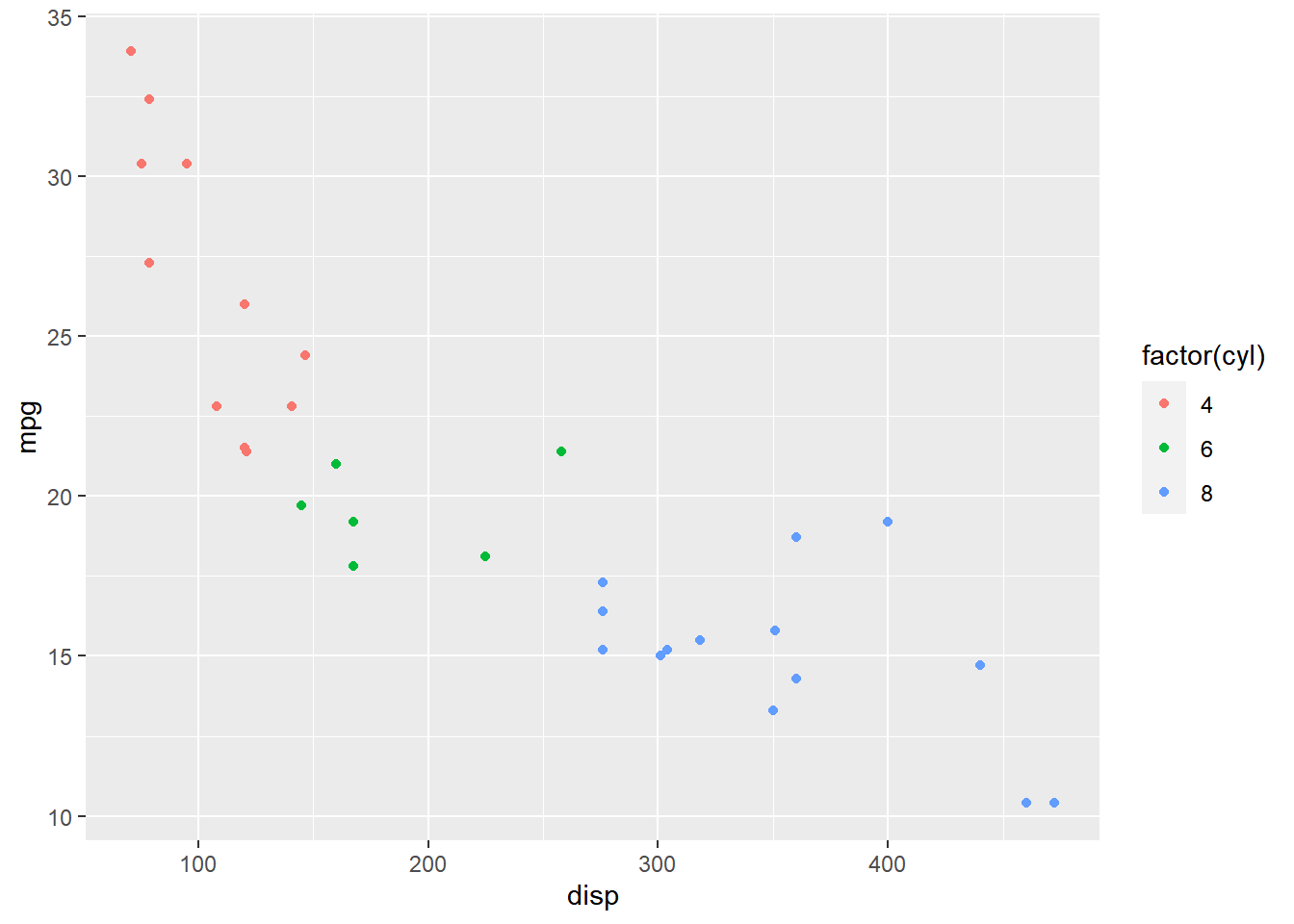# Articles by Rsquared Academy Blog

### Data Wrangling with dbplyr

December 8, 2018 |

Introduction This is the second post in the series R & Databases. You can find the links to the first post of this series below: Quick Guide: R & SQLite In this post, we will learn to query data from a database using dplyr. Libraries, Code & Data We will use the following ... [Read more...]

### Quick Guide: R & SQLite

November 26, 2018 |

Introduction This is the first post in the series R & Databases. You can find the links to the other two posts of this series below: Data Wrangling with dbplyr SQL for Data Science - Part 1 SQL for Data Science - Part 2 In this post, we will learn to: connect to ... [Read more...]

### Categorical Data Analysis using forcats

November 14, 2018 |

Introduction In this post, we will learn to work with categorical/qualitative data in R using forcats. Let us begin by installing and loading forcats and a set of other pacakges we will be using. Libraries & Code We will use the following packages: forcats dplyr magrittr ggplot2 tibbe purrr and ...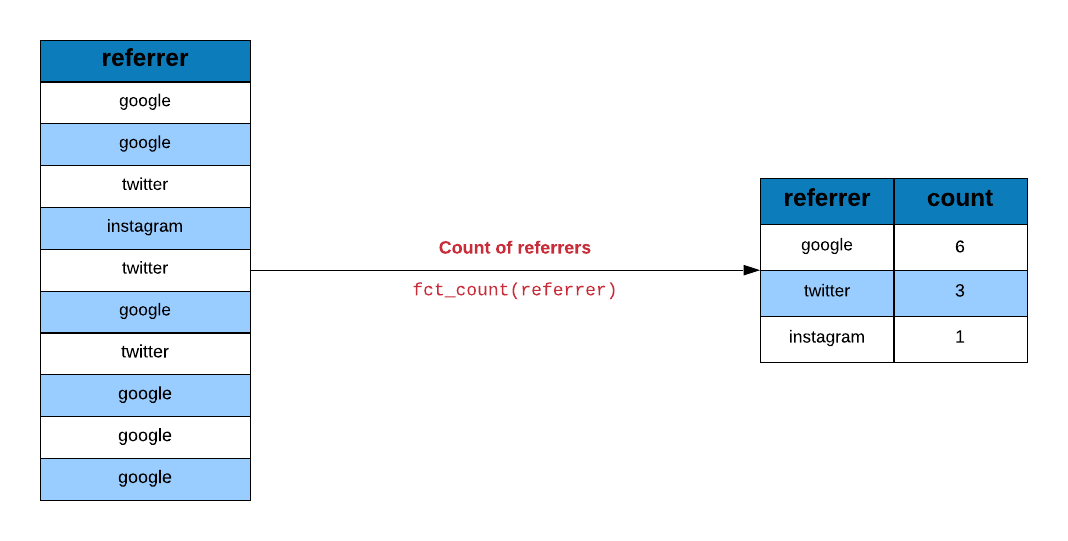### Working with Date and Time in R

November 2, 2018 |

Introduction In this post, we will learn to work with date/time data in R using lubridate, an R package that makes it easy to work with dates and time. Let us begin by installing and loading the pacakge. Libraries, Code & Data We will use the following packages: lubridate dplyr ...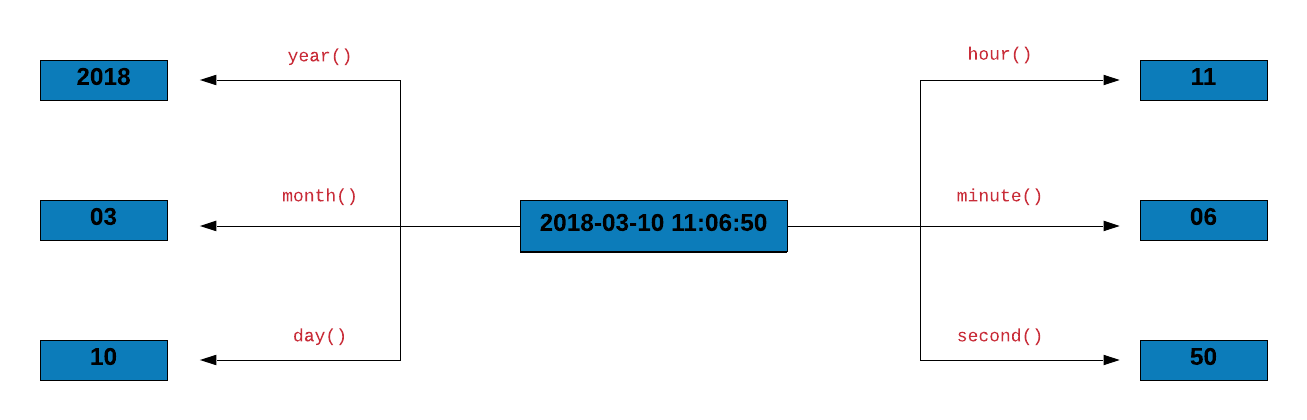### Hacking strings with stringr

October 21, 2018 |

Introduction In this post, we will learn to work with string data in R using stringr. As we did in the other posts, we will use a case study to explore the various features of the stringr package. Let us begin by installing and loading stringr and a set of ...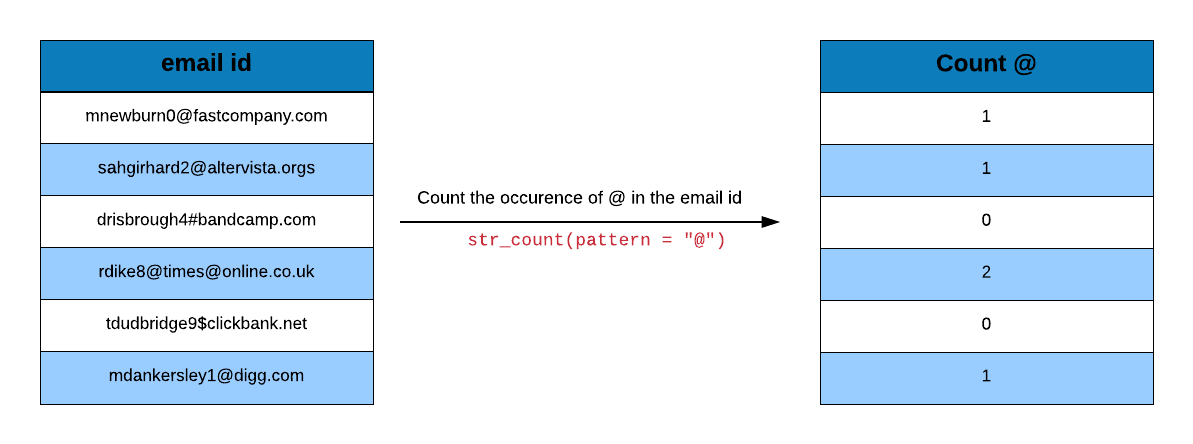October 9, 2018 |

Introduction R code contain a lot of parentheses in case of a sequence of multiple operations. When you are dealing with complex code, it results in nested function calls which are hard to read and maintain. The magrittr package by Stefan Milton Bache provides pipes enabling us to write R ...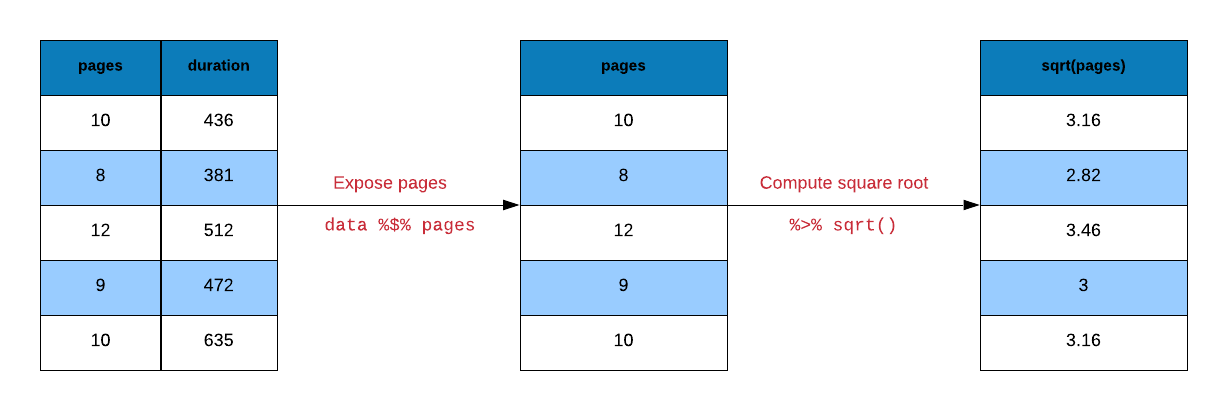### Introduction to tibbles

September 27, 2018 |

Introduction A tibble, or tbl_df, is a modern reimagining of the data.frame, keeping what time has proven to be effective, and throwing out what is not. Tibbles are data.frames that are lazy and surly: they do less (i.e. they don’t change variable names or types, ... [Read more...]

### Data Wrangling with dplyr – Part 3

September 15, 2018 |

Introduction In the previous post, we learnt to combine tables using dplyr. In this post, we will explore a set of helper functions in order to: extract unique rows rename columns sample data extract columns slice rows arrange rows compare tables extract/mutate data using predicate functions count observations for ...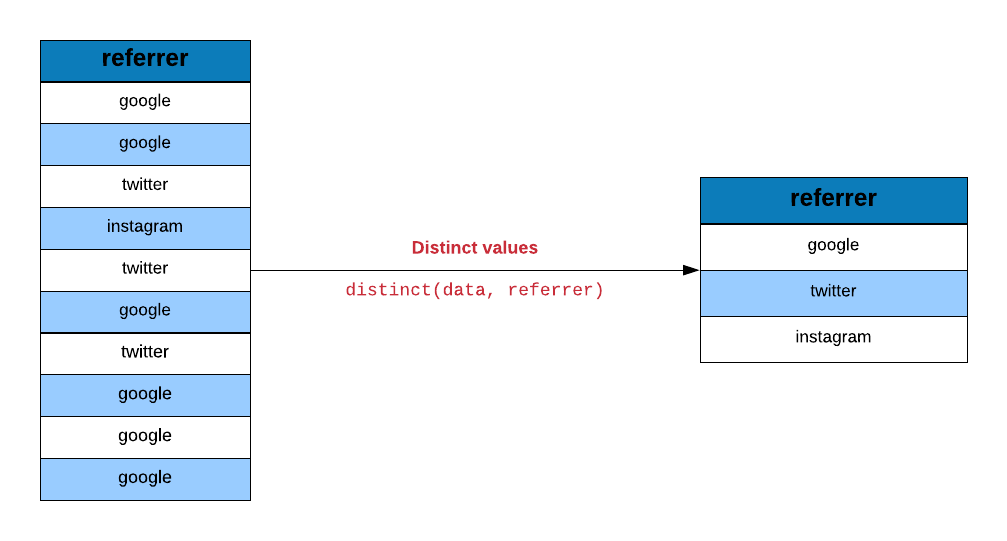### Data Wrangling with dplyr – Part 2

September 3, 2018 |

Introduction In the previous post we learnt about dplyr verbs and used them to compute average order value for an online retail company data. In this post, we will learn to combine tables using different *_join functions provided in dplyr. Libraries, Code & Data We will use the following packages: dplyr ...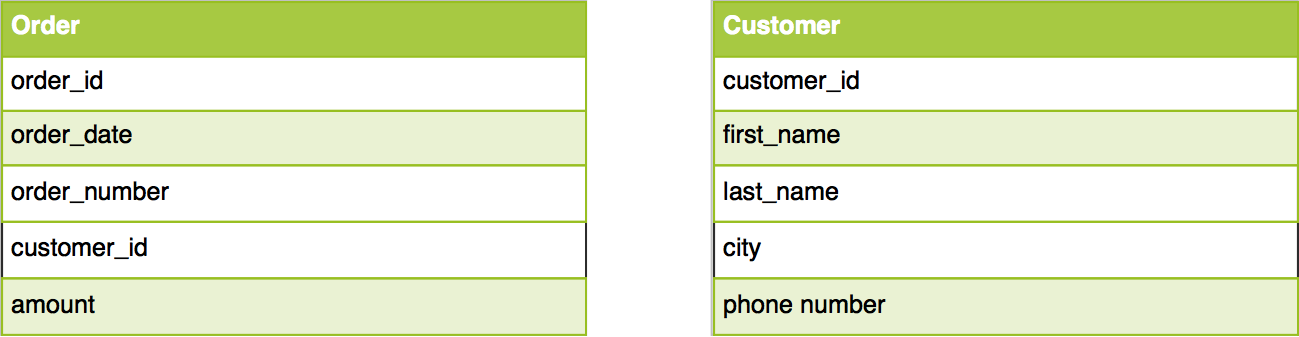### Data Wrangling with dplyr – Part 1

August 22, 2018 |

Introduction According to a survey by CrowdFlower, data scientists spend most of their time cleaning and manipulating data rather than mining or modeling them for insights. As such, it becomes important to have tools that make data manipulation faster and easier. In today’s post, we introduce you to dplyr, ...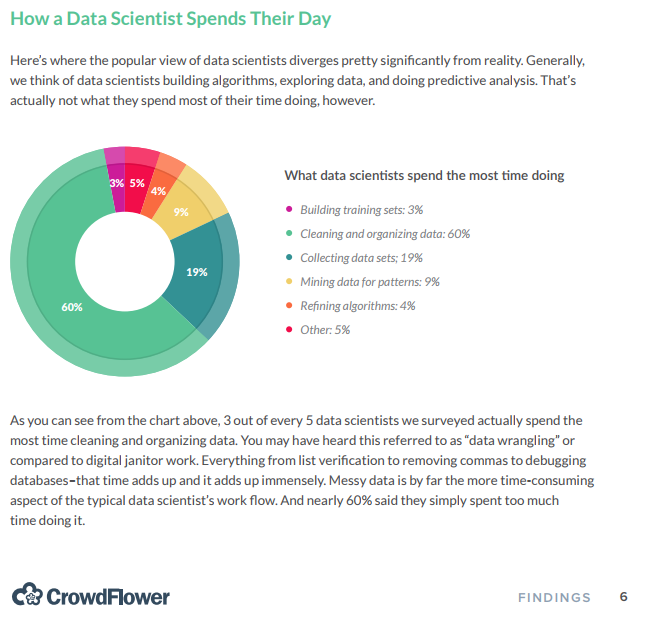### Import Data into R – Part 2

August 10, 2018 |

Introduction This is the second post in the series Importing Data into R. In the previous post, we explored reading data from flat/delimited files. In this post, we will: list sheets in an excel file read data from an excel sheet read specific cells from an excel sheet read ...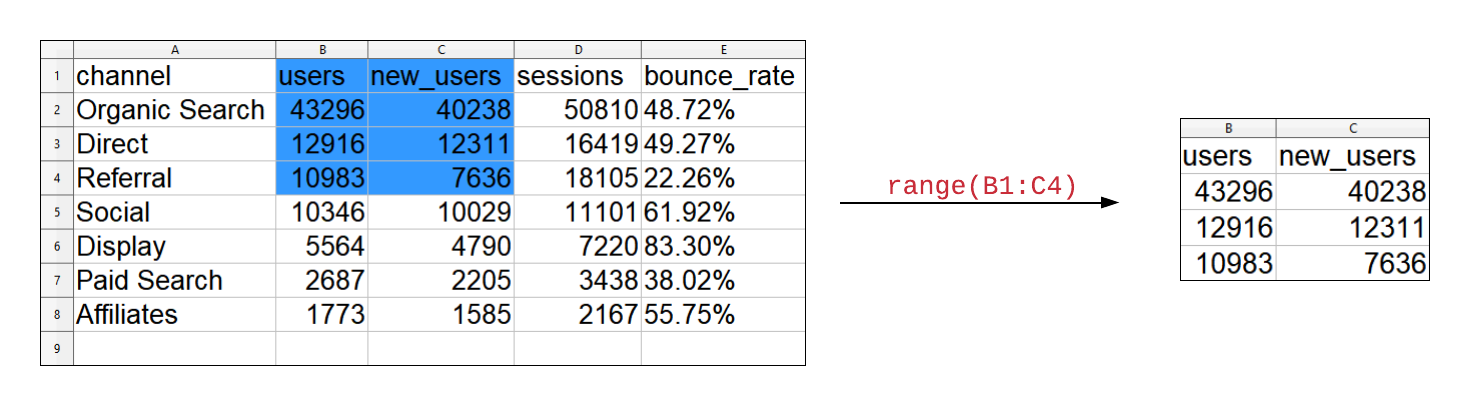### Import Data into R – Part 1

July 29, 2018 |

Introduction In this post, we will learn to: read data from flat or delimited files handle column names/header skip text/info present before data specify column/variable types read specific columns/variables Libraries, Data & Code We will use the readr package. The data sets can be downloaded from here ...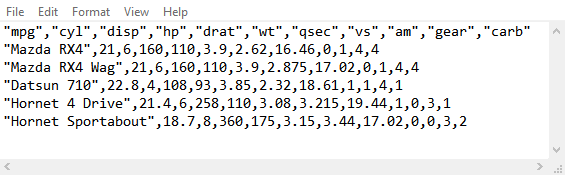### ggplot2: Themes

May 6, 2018 |

Introduction This is the last post in the series Elegant Data Visualization with ggplot2. In the previous post, we learnt to combine multiple plots. In this post, we will learn to modify the appearance of all non data components of the plot such as: axis legend panel plot area background ...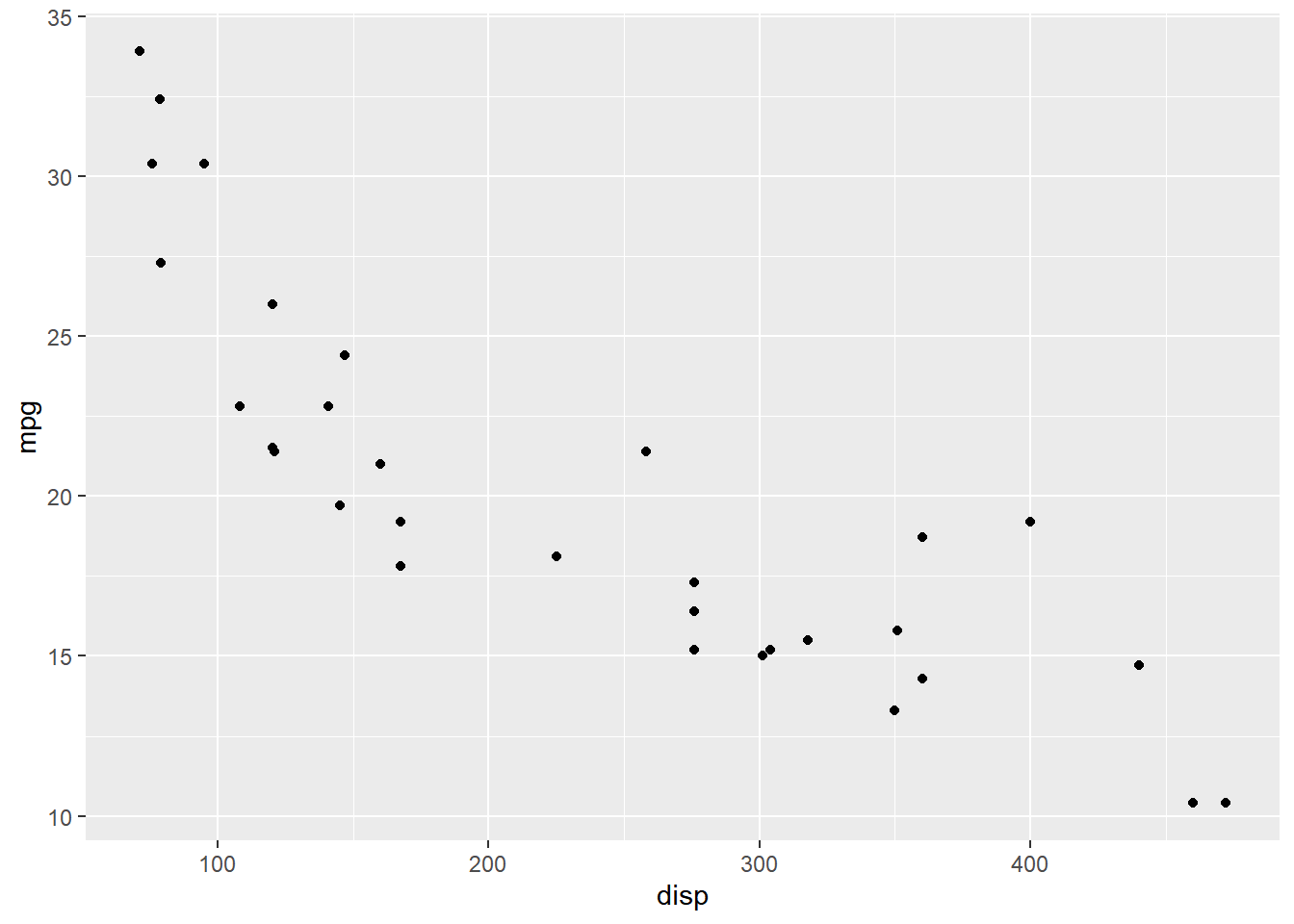### ggplot2: Faceting

April 24, 2018 |

Introduction This is the 19th post in the series Elegant Data Visualization with ggplot2. In the previous post, we learnt to modify the title, label and bar of a legend. In this post, we will learn about faceting i.e. combining plots. Libraries, Code & Data We will use the following ...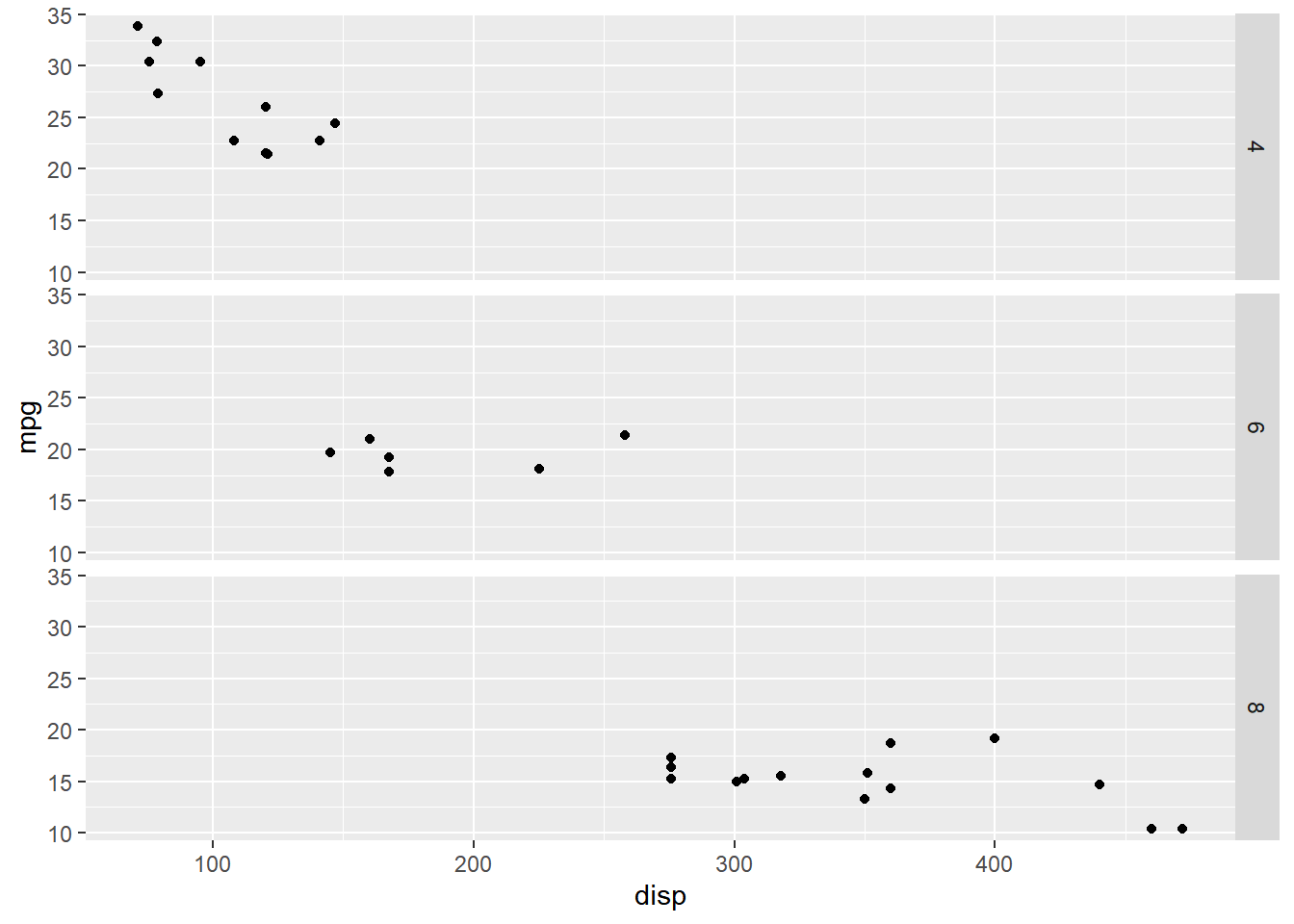### ggplot2: Legend – Part 6

April 12, 2018 |

Introduction This is the 18th post in the series Elegant Data Visualization with ggplot2. In the previous post, we learnt how to modify the legend of plot when alpha is mapped to a categorical variable. In this post, we will learn to modify legend title label and bar So far, ...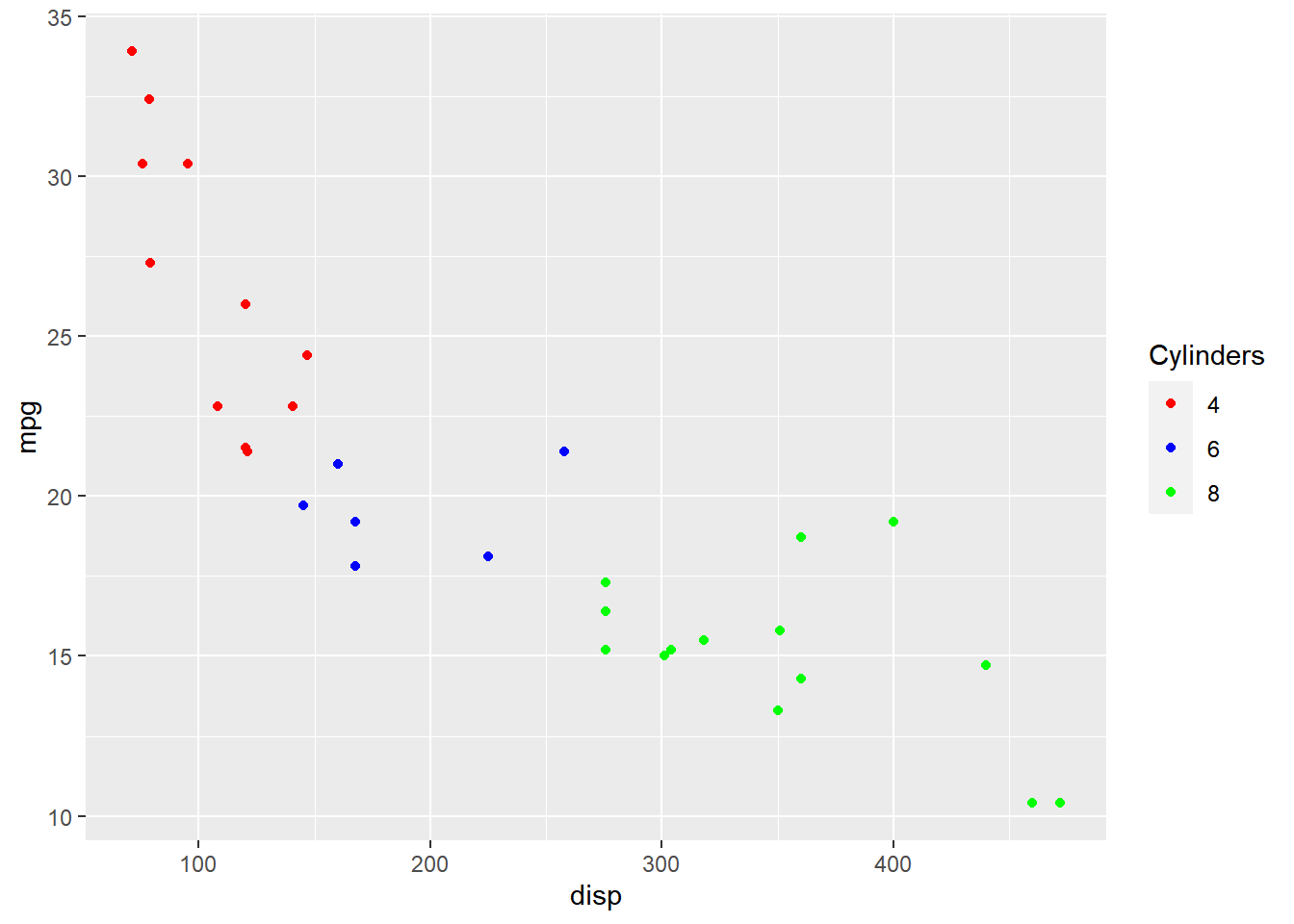### ggplot2: Legend – Part 5

March 31, 2018 |

Introduction This is the 17th post in the series Elegant Data Visualization with ggplot2. In the previous post, we learnt how to modify the legend of plot when size is mapped to continuous variable. In this post, we will learn to modify the following using scale_alpha_continuous() when alpha ...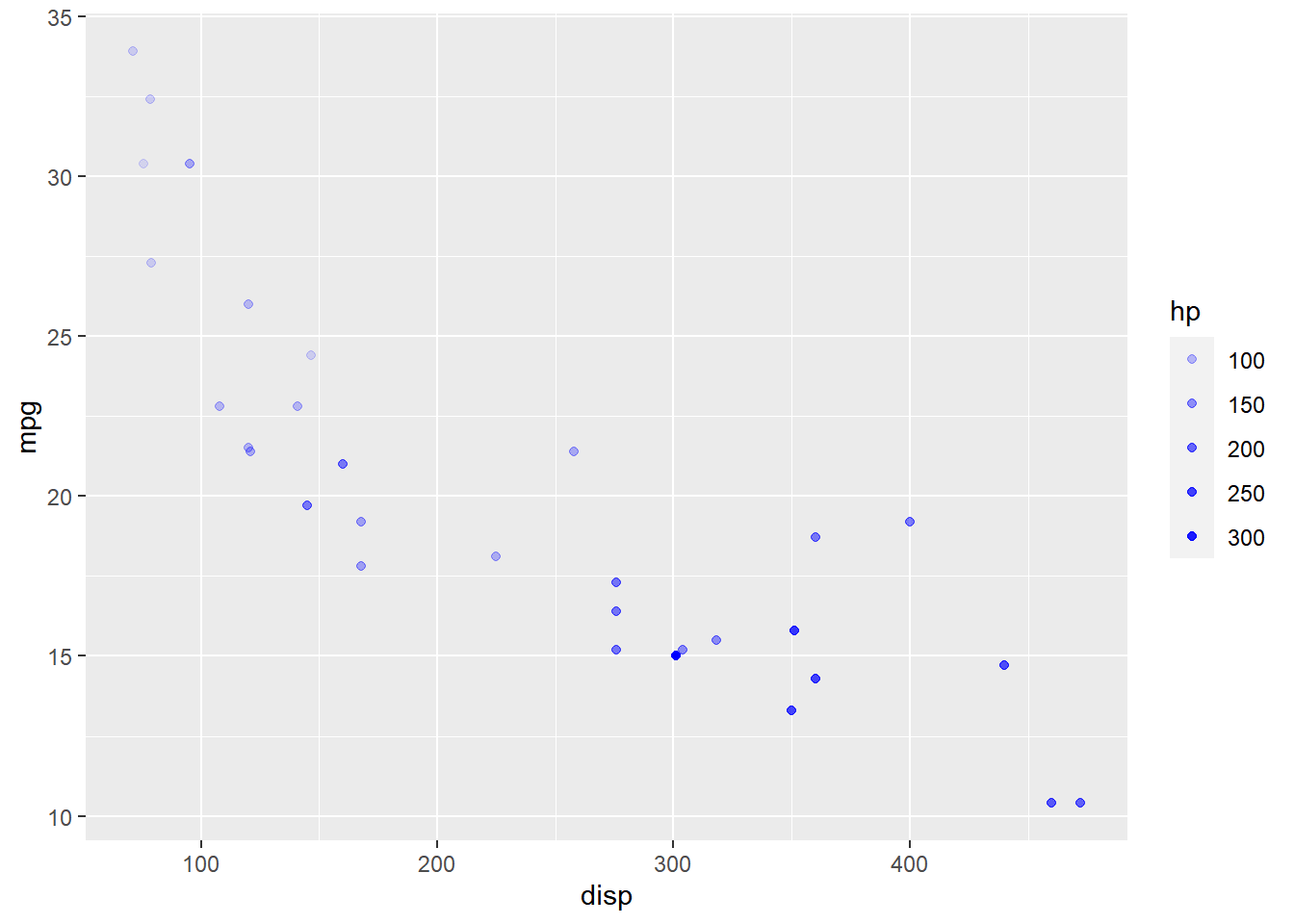### ggplot2: Legend – Part 4

March 19, 2018 |

Introduction This is the 16th post in the series Elegant Data Visualization with ggplot2. In the previous post, we learnt how to modify the legend of plot when shape is mapped to categorical variables. In this post, we will learn to modify the following using scale_size_continuous when size ...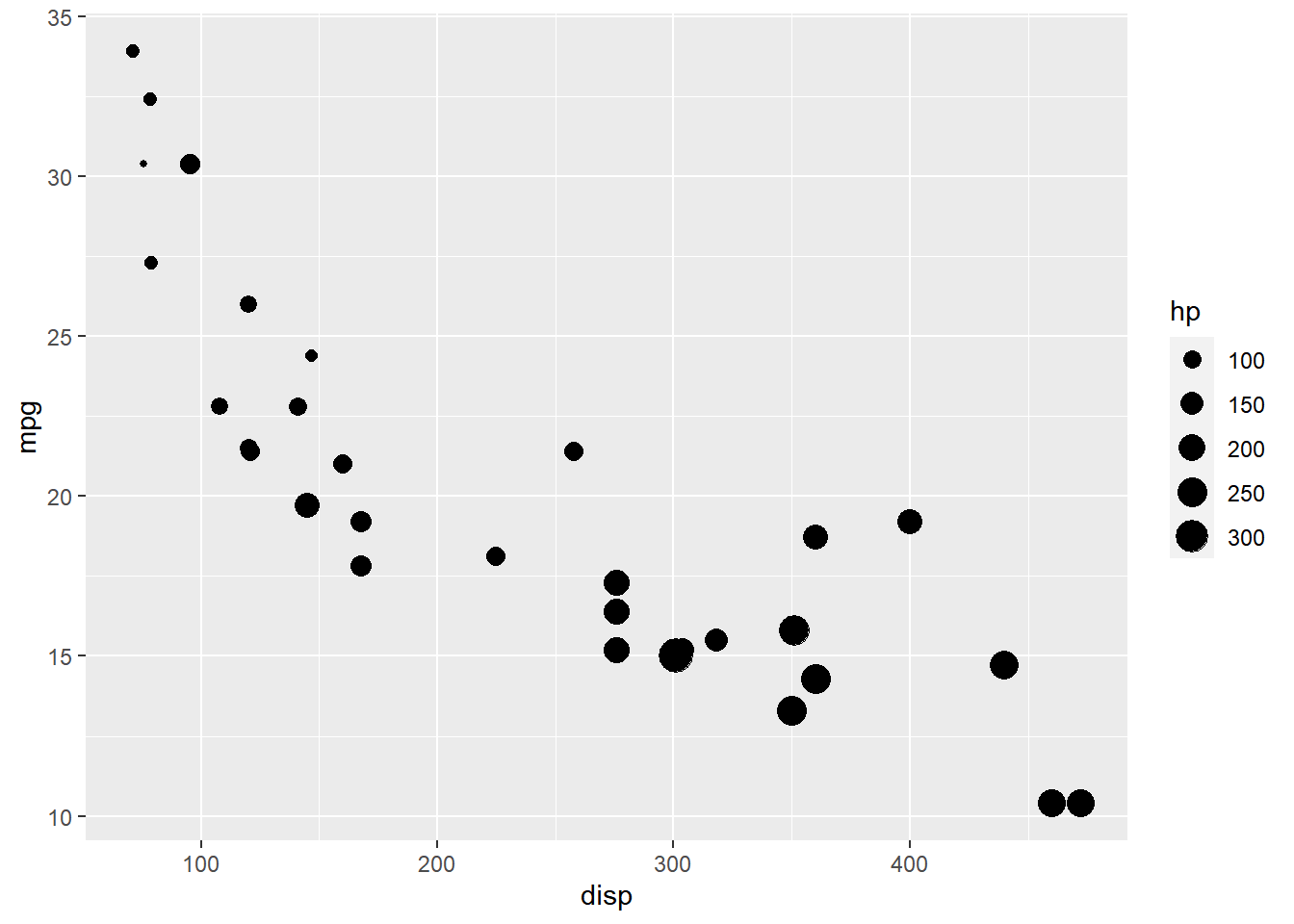### ggplot2: Legend – Part 3

March 7, 2018 |

Introduction This is the 15th post in the series Elegant Data Visualization with ggplot2. In the previous post, we learnt how to modify the legend of plots when aesthetics are mapped to variables..In this post, we will learn to modify the following using scale_shape_manual when shape is ...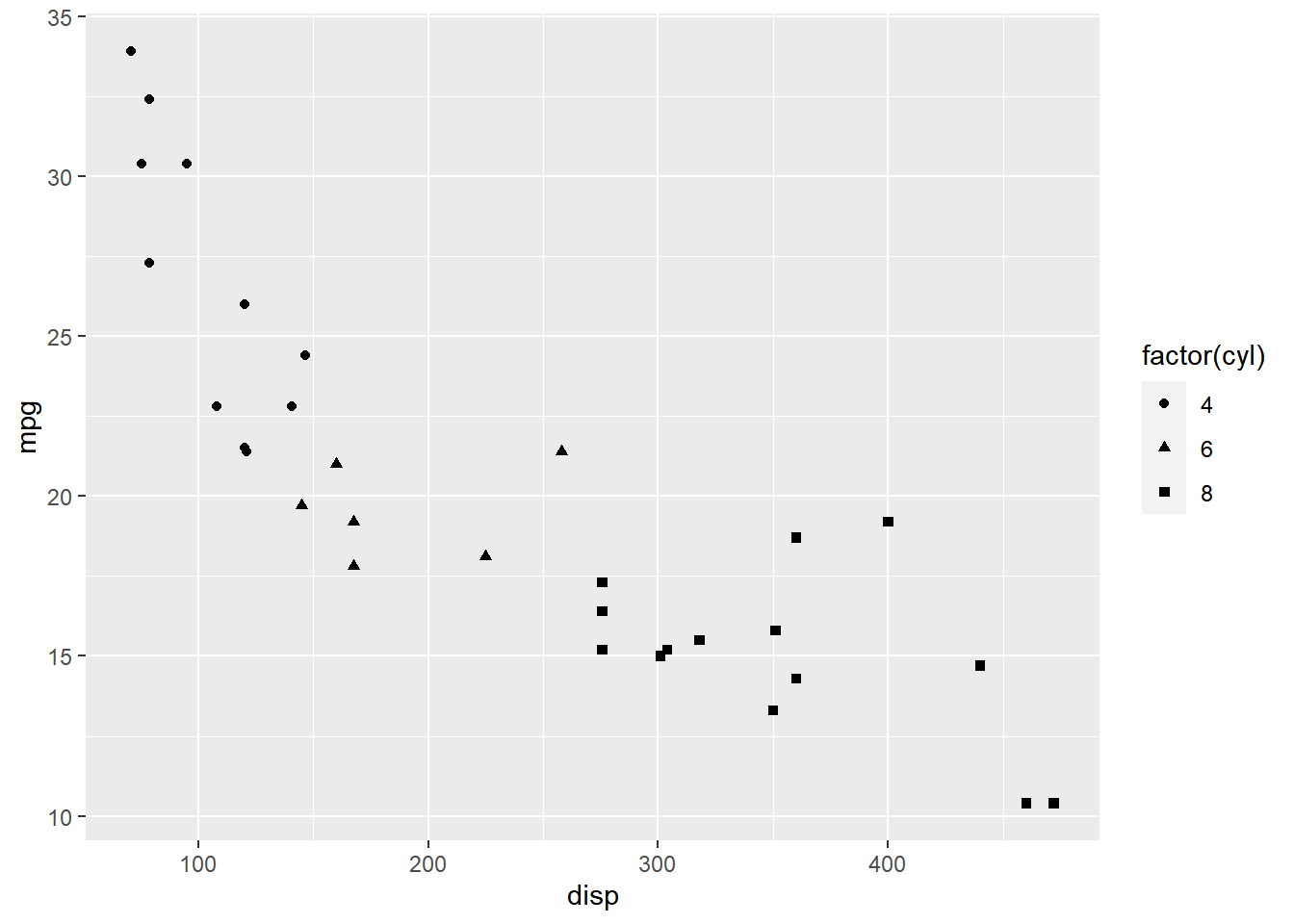### ggplot2: Legend – Part 2

February 23, 2018 |

Introduction This is the 14th post in the series Elegant Data Visualization with ggplot2. In the previous post, we learnt how to modify the legend of plots when aesthetics are mapped to variables. In this post, we will continue to explore different ways to modify/customize the legend of plots. ...